﻿ Rule of 78
WinAmort Professional 2.0

Rule of 78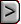Rule of 78Rule of 78The set-up of the Rule of 78 screen is identical to that of the Normal loan type.

Even the calculations on the form will be the same. The difference arises when

an amortization table is created. In a Rule of 78 loan, a larger amount of interest

is charged at the beginning of the loan and this amount decreases as the loan

progresses. The total interest charge is the same as that for a Normal loan. In

cases where a loan is paid off early, the lender benefits because of the higher

interest charges at the beginning.

 Rule of 78 - EXAMPLE You want to purchase a car for \$20,000.00 with a down payment of \$2,000.00. The car dealer will finance the rest of the purchase price with a Rule of 78 loan for a term of 4 years at 13.25% interest compounded monthly. The purchase date is December 27, 2010 and the first payment is due one month later. What is the monthly payment? How much is the interest and principal at the end of the first year? How does this compare to the Normal loan type?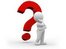Solve The Payment amount.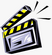Enter the data into the form as given.

 Type       Type     Type   Type       Type 12-27-2011 for the Origination Date. This is the date on which the Loan is taken out.   01/27/2011 for the First Payment Date. This is the date on which the first payment on the mortgage will be made. This date can also be computed automatically by pressing the Compute button beside the field.   18000 in the Principal field. ( \$20,000 less the \$2000 down payment.)   13.25 in the Interest Rate field. The interest rate that you enter should always be the annual interest rate. Note that the Effective Rate will automatically be calculated and displayed (see Glossary for definition).   4 - in the Period field. (This is in Years)   Leave the Balloon Payment amount at zero since we don’t need it for  this example.

 Press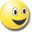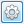button beside the Payment field to compute the answer.     The monthly payment is \$485.13.

Your form should look like this.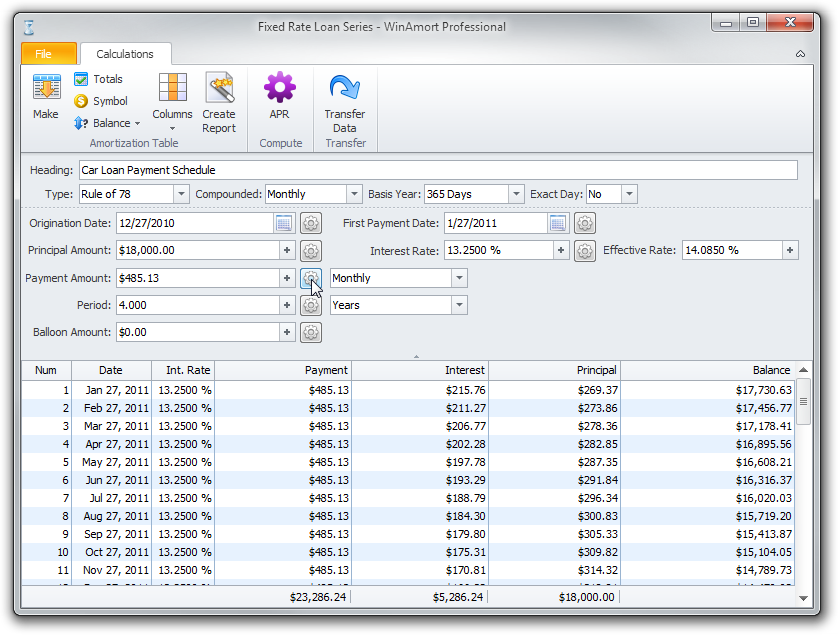The table is automatically generated when you click the Payment Amount Compute button.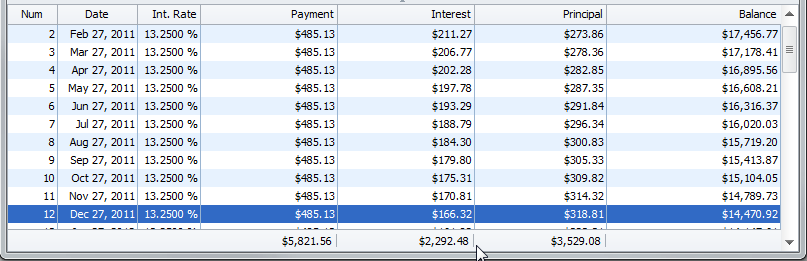Scroll down the table using the side bar or the wheel on your mouse and click on Dec. 27, 2012 (this is line number 12) and notice, that the total interest for the first year is \$2,292.48 and principal balance is \$14,470.92.

Now let's compare these values with a REGULAR LOAN type, by generating another table.

Change the Option for loan type from RULE OF 78 to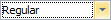Pressbutton to regenerate the table using the REGULAR Loan type parameters.

Once the NEW table is generated again, scroll down to line 12 again and click on it.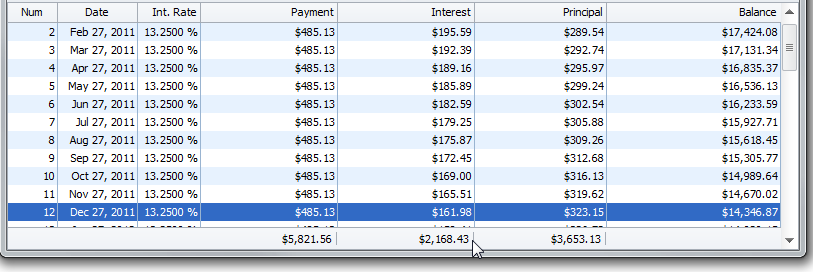This time, the first year’s interest for this table is \$2,168.43 and the principal balance is \$14,346.87.

When you compare these values to the Rule of 78 values, we had first year interest of \$2,292.48 and a principal balance of \$14,470.92 .

The difference between the Normal loan type and the Rule of 78 loan type is that \$124.05 more interest is paid in the Rule of 78 loan. Although, if you compare the total interest cost at the end of the loan for both loan types, it will be equal.

What this shows is that the Rule of 78 Loan type has been designed to collect more interest earlier or up front and less interest towards the end of the amortization period. There is no difference to the borrower or the lender if the loan is paid regularly up to the end of the amortization period. However, if the borrower decides to pay off the loan earlier, the Rule of 78 loan benefits the lender because more interest has been collected early.

Goto: Fixed Principal